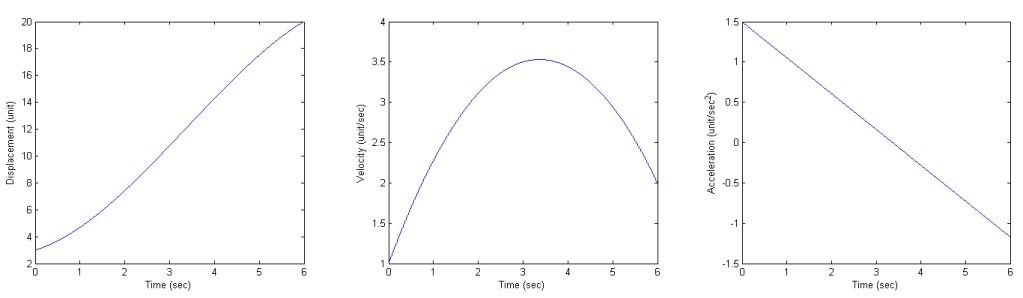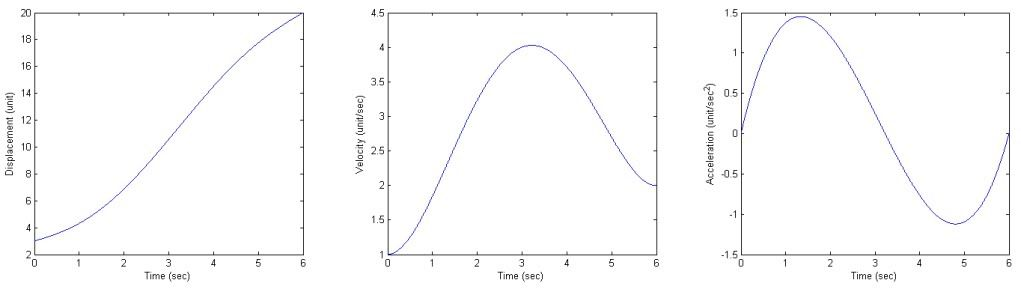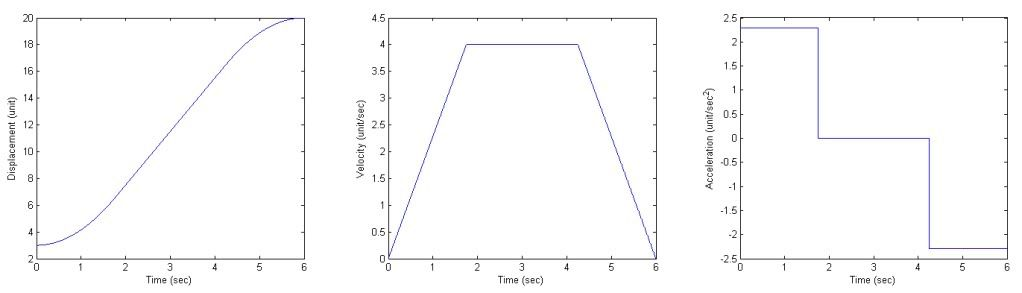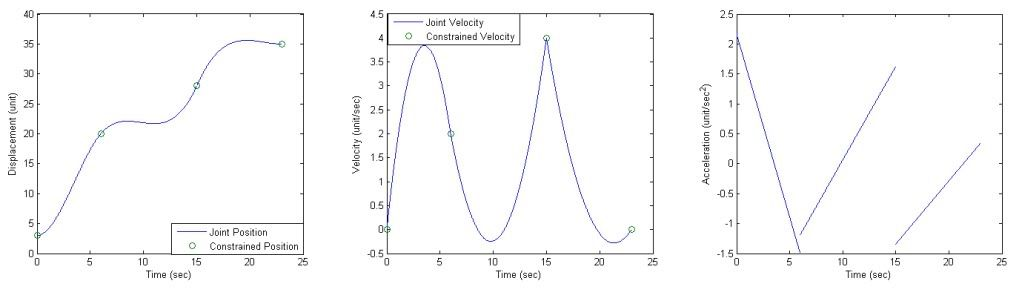Trajectory Planning for Point to Point Motion

# Introduction

Trajectory planning for point to point motion maps position as function of time between specified points. Velocity and acceleration along the trajectory can be computed by differentiating position with respect to time, and for a smooth path, velocity cannot have any discontinuities or the specified trajectory would require infinite acceleration. With a given set of via points and constraints, a smooth trajectory ($q(t)$) can be specified using several methods.

# Cubic Polynomial Trajectories

Cubic Polynomial Trajectories defines the path between two points ($q(t_0)$ and $q(t_f)$) with a cubic polynomial.

(1)
\begin{equation} q(t) = a_0+a_1t+a_2t^2+a_3t^3 \end{equation}

Differentiating Equation 1, velocity and acceleration can be calculated.

(2)
\begin{align} \dot q(t) = v(t)=a_1+2a_2t+3a_3t^2 \end{align}
(3)
\begin{align} \ddot q(t) = \alpha(t)=2a_2+6a_3t \end{align}

Equation 3 shows that acceleration linearly varies with time and is continuous; thus, the trajectory does not require infinite accelerations. Four constraints must be specified to solve the unknowns: $a_0$, $a_1$, $a_2$, and $a_3$. Obviously, two constraints are the start and end positions, and the final two are the initial and end velocities.

(4)
\begin{equation} q(t_0) = a_0+a_1t_0+a_2t_0^2+a_3t_0^3 \end{equation}
(5)
\begin{equation} v(t_0)=a_1+2a_2t_0+3a_3t_0^2 \end{equation}
(6)
\begin{equation} q(t_f) = a_0+a_1t_f+a_2t_f^2+a_3t_f^3 \end{equation}
(7)
\begin{equation} v(t_f)=a_1+2a_2t_f+3a_3t_f^2 \end{equation}

Equations 4-7 can be combined into one matrix equation.

(8)
\begin{align} \left[\begin{array}{cccc} 1 & t_0 &t_0^2 &t_0^3 \\ 0 & 1 & 2t_0 & 3t_0^2 \\ 1 & t_f &t_f^2 &t_f^3 \\ 0 & 1 & 2t_f & 3t_f^2 \end{array} \right] \left[\begin{array}{c} a_0\\ a_1\\a_2\\a_3 \end{array}\right] = \left[ \begin{array}{c} q_0\\v_0\\q_f\\v_f \end{array} \right] \end{align}Figure 1: Cubic Polynomial Trajectory with: $t_0= 0$, $t_f= 0$, $q_0= 3$, $q_f= 20$, $v_0= 1$, and $v_f= 2$.

# Quintic Polynomial Trajectories

Note that the accelerations cannot be specified at each point using the Cubic Polynomial Trajectory method, and for a set of points, acceleration will be discontinuous at each point. This discontinuity of acceleration makes the derivative of acceleration (jerk) infinite at each via point, and causes an impulsive jerk in the motion of the robot. To avoid this, three constraints at each point must be specified: position, velocity, and acceleration. Therefore, a fifth order polynomial is required to define the trajectory between two via points.

(9)
\begin{equation} q(t) = a_0+a_1t+a_2t^2+a_3t^3+a_4t^4+a_5t^5 \end{equation}

Therefore, the constraints at points $q(t_0)$ and $q(t_f)$ can be defined as follows.

(10)
\begin{equation} q(t_0) = a_0+a_1t_0+a_2t_0^2+a_3t_0^3+a_4t_0^4+a_5t_0^5 \end{equation}
(11)
\begin{equation} v(t_0)=a_1+2a_2t_0+3a_3t_0^2+4a_4t_0^3+5a_5t_0^4 \end{equation}
(12)
\begin{align} \alpha(t_0)=2a_2+6a_3t_0+12a_4t_0^2+20a_5t_0^3 \end{align}
(13)
\begin{equation} q(t_f) = a_0+a_1t_f+a_2t_f^2+a_3t_f^3+a_4t_f^4+a_5t_f^5 \end{equation}
(14)
\begin{equation} v(t_f)=a_1+2a_2t_f+3a_3t_f^2+4a_4t_f^3+5a_5t_f^4 \end{equation}
(15)
\begin{align} \alpha(t_f)=2a_2+6a_3t_f+12a_4t_f^2+20a_5t_f^3 \end{align}

Equations 10-15 can be put into matrix form.

(16)
\begin{align} \left[\begin{array}{cccccc} 1 & t_0 &t_0^2 &t_0^3 & t_0^4 &t_0^5\\ 0 & 1 & 2t_0 & 3t_0^2 & 4t_0^2 & 5t_0^4 \\ 0 & 0 & 2 & 6t_0 & 12t_0^2 & 20t_0^3 \\ 1 & t_f &t_f^2 &t_f^3 & t_f^4 &t_f^5\\ 0 & 1 & 2t_f & 3t_f^2 & 4t_f^2 & 5t_f^4 \\ 0 & 0 & 2 & 6t_f & 12t_f^2 & 20t_f^3 \end{array} \right] \left[ \begin{array}{c} a_0\\a_1\\a_2\\a_3\\a_4\\a_5 \end{array} \right] = \left[ \begin{array}{c} q_0 \\ v_0 \\ \alpha_0 \\ q_f \\ v_f \\ \alpha_f \end{array} \right] \end{align}Figure 2: Quintic Polynomial Trajectory with: $t_0= 0$, $t_f= 0$, $q_0= 3$, $q_f= 20$, $v_0= 1$, $v_f= 2$, $\alpha_0= 0$, and $\alpha_f= 0$.

# Linear Segments with Parabolic Blends (LSPB)

When a constant velocity ($V$) for a portion of a trajectory is desired, the LSPB path planning method can be implemented. Velocity ramps up from the start time to the blend time ($t_b$) ; holds constant velocity form ($t_b$) to $t_f-t_b$; and finally decelerates from $t_f-t_b$ to $t_f$ . This makes a trapezoidal velocity profile, and position can be defined as:

(17)
\begin{align} q(t) = \left\{ \begin{array}{cc} a_0+a_1t+a_2t^2 & t_0 \eq t \leq t_b \\ Vt +c & t_b < t \leq (tf-t_b) \\ \bar a_0+ \bar a_1t+ \bar a_2t^2 & (t_f-t_b) < t \leq t_f \end{array} \right \end{align}

To derive the equations a LSPB trajectory, it will be assumed that $t_0 = 0$ and $\dot q(0)= \dot q(t_f) = 0.$. With these assumptions, it can be shown that:

(18)
\begin{equation} a_0 = q_0 \end{equation}
(19)
\begin{equation} a_1=0 \end{equation}

At the blend time, the desired velocity, $V$, must be reached. Therefore, $\dot q(t_b) = 2a_2t_b = V$ which gives

(20)
\begin{align} a_2 = \frac{V}{2t_b}=\frac{\alpha}{2} \end{align}

Because,

(21)
\begin{align} \alpha = \frac{V}{t_b} \end{align}

For the constant velocity section, position can be defined by:

(22)
\begin{equation} q(t) = q(t_b) + V(t-t_b) \end{equation}

Knowing that the trapezoidal profile is symmetric about $\frac{t_f}{2}$, it can be shown that

(23)
\begin{align} t_b = \frac{q_0-q_f+Vt_f}{V} \end{align}

Now substituting Equation 23 into Equation 22, gives

(24)
\begin{align} c = \frac{q_f+q_0-Vt_f}{2} \end{align}

Now, the trajectory for the final deceleration region must be derived. Knowing $\dot q(t_f) =0$ and $q(t_f) = q_f$, the following holds true for $(t_f-t_b) < t \leq t_f$.

(25)
\begin{align} \dot q(t) = \alpha (t_f-t) \end{align}
(26)
\begin{align} q(t) = q_f-\frac{\alpha t_f^2}{2} +\alpha t_f t-\frac{\alpha}{2} t^2 \end{align}

Finally, the derived equations can be substituted into Equation 17.

(27)
\begin{align} q(t) = \left\{ \begin{array}{cc} q_0+\frac{\alpha}{2}t^2 & t_0 \eq t \leq t_b \\ Vt +\frac{q_f+q_0-Vt_f}{2} & t_b < t \leq (tf-t_b) \\ q_f-\frac{\alpha t_f^2}{2} +\alpha t_f t-\frac{\alpha}{2} t^2 & (t_f-t_b) < t \leq t_f \end{array} \right \end{align}Figure 3: LSPB Trajectory with: $V= 4$, $t_f= 6$, $t_b= 1.75$, and $\alpha = 2.29$.

# Trajectories for Paths Specified by Via Points

Previously, a few methods were outlined for planning a trajectory between two points. Now, consider a set of via points and desired velocities at each point. The Cubic Polynomial Trajectory method can be used to map a trajectory to meet specified constraints. Note that for n via points, there will be n-1 cubic polynomials and 2n constraints to describe the desired path. At every point, time ($t$), position ($q$), and velocity ($v$) must be known.Figure 4: Cubic Polynomial Trajectory with 4 via points.

Bibliography
1. Spong, Hutchinson, and Vidyasagar, Robot Modeling and Control